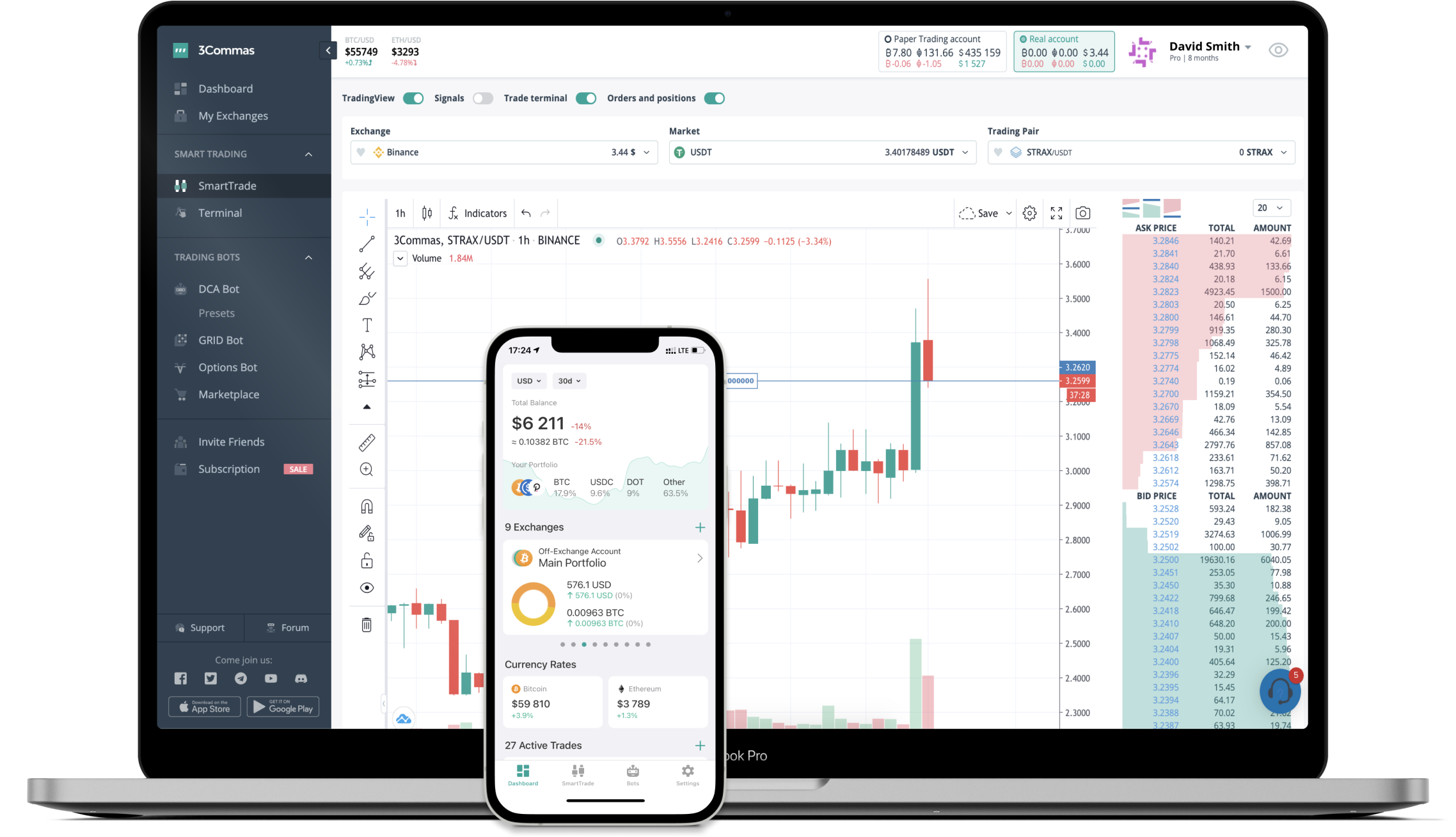# DELTA to JPY Converter

Delta Financial
PriceJPY 161.18-1.69%
VolumeJPY 341,241
How it work

The 3Commas currency calculator allows you to convert a currency from Delta Financial (DELTA) to Japanese yen (JPY) in just a few clicks at live exchange rates.

Simply enter the amount of Delta Financial you wish to convert to JPY and the conversion amount automatically populates. You can also use our Prices Calculator Table to calculate how much your currency is worth in other denominations, i.e. .1 DELTA, .5 DELTA, 1 DELTA, 5 DELTA, or even 10 DELTA.

## DELTA to JPY Exchange Calculator

The chart displays Delta Financial price changes in JPY.

The ultimate tools for crypto traders to maximize trading profits while minimizing risk and loss.## Latest DELTA to JPY price calculator

Delta Financial market price is updated every three minutes and is automatically displayed in JPY. Below are the most popular denominations to convert to JPY.

Delta Financial
Japanese yen
1.61
JPY
16.12
JPY
161.18
JPY
322.36
JPY
483.54
JPY
805.90
JPY
1611.80
JPY
4029.50
JPY
8059.00
JPY
16118.00
JPY
40295.00
JPY
80590.00
JPY
161180.00
JPY
402950.00
JPY
Japanese yen
Delta Financial
0.00006204
DELTA
0.00062042
DELTA
0.00620424
DELTA
0.01240849
DELTA
0.01861273
DELTA
0.03102122
DELTA
0.06204244
DELTA
0.15510609
DELTA
0.31021219
DELTA
0.62042437
DELTA
1.55106093
DELTA
3.10212185
DELTA
6.20424370
DELTA
15.51060926
DELTA

## FAQ

### How much is 1 Delta Financial in Japanese yen?

Delta Financial price in JPY is constantly changing.

At this moment, 1 Delta Financial equals 161.18 JPY

### How do I calculate the conversion from DELTA to JPY?

The 3Commas Delta Financial Calculator allows you to easily calculate the conversion price of DELTA to JPY by simply entering the amount of Delta Financial in the corresponding field and will automatically convert the value in Japanese yen (JPY).

You can also use our Delta Financial price table above to check the latest Delta Financial price in major fiat and crypto currencies.

### How can I convert Delta Financial to JPY?

The most common way of converting DELTA to JPY is by using a Crypto Exchange or a P2P (person-to-person) exchange platform like LocalBitcoins, etc.